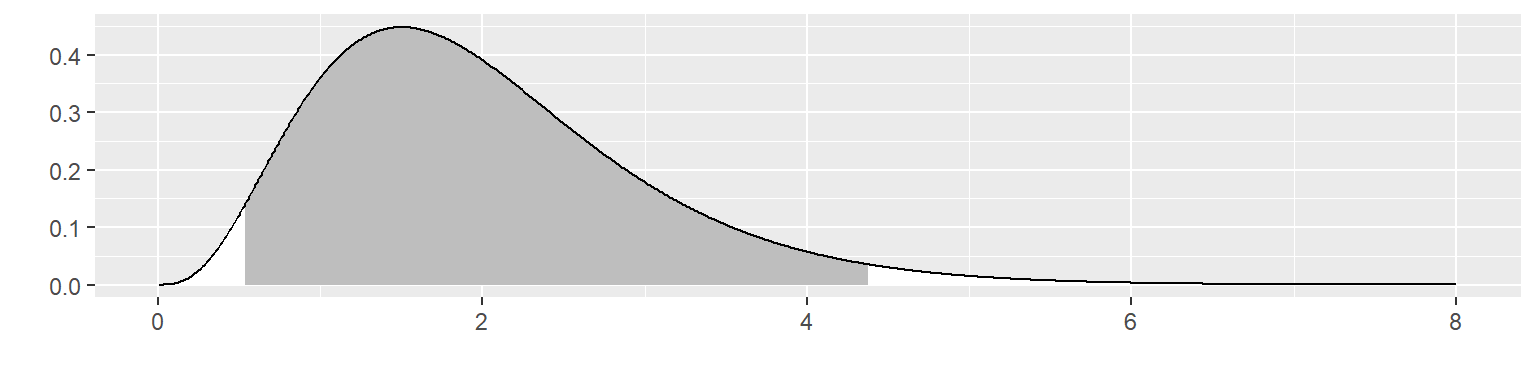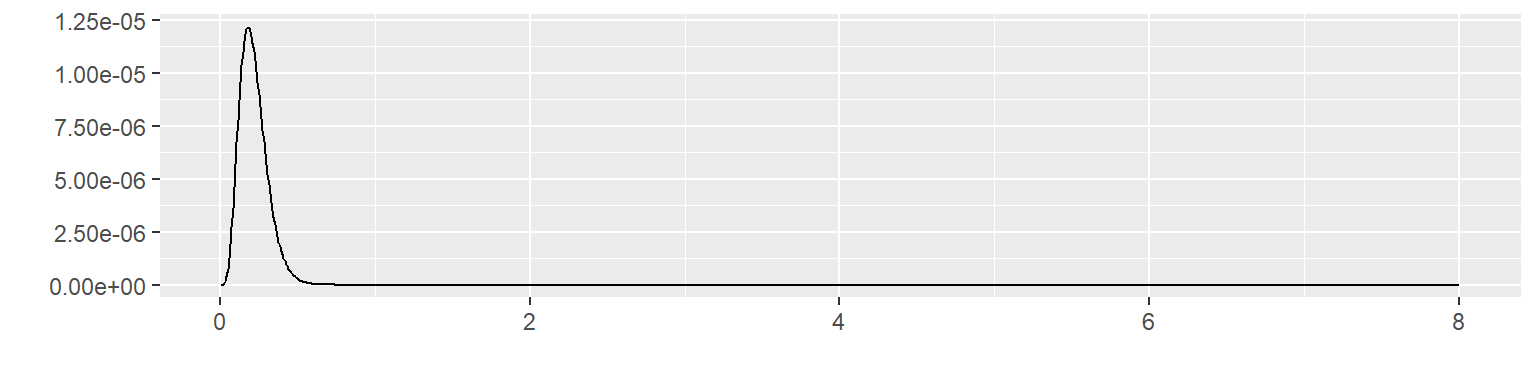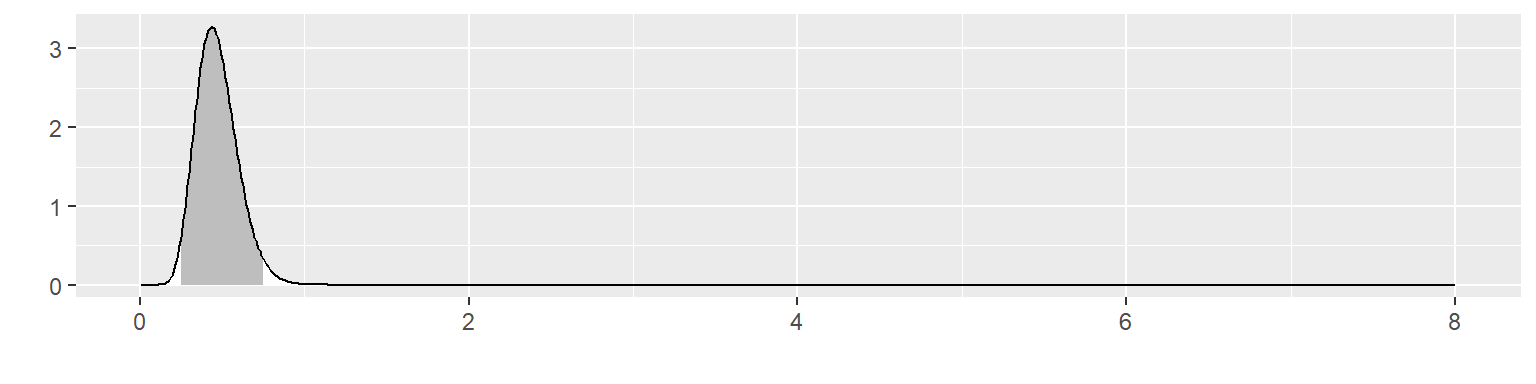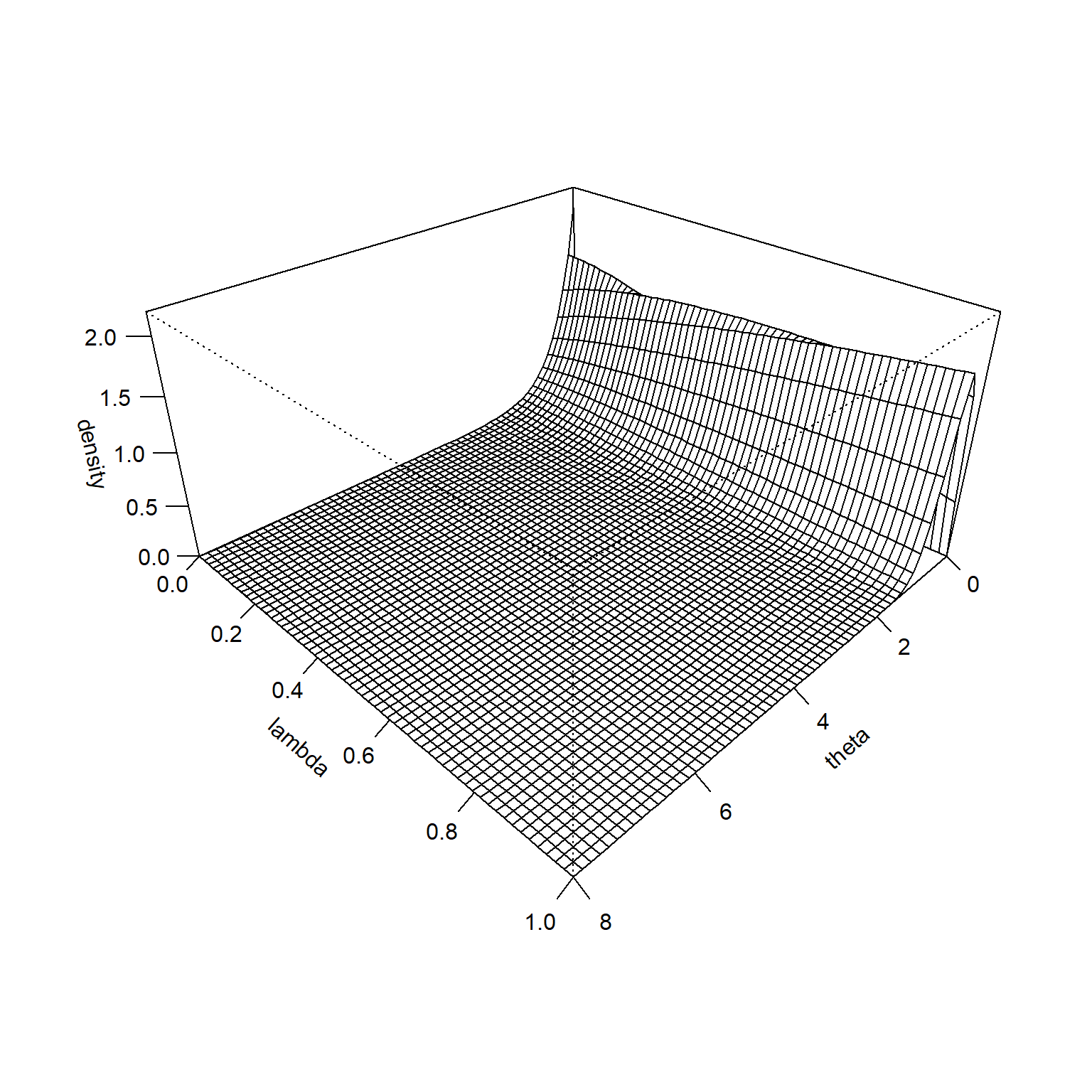# The hedging hyperprior for the normal distribution: unknown mean, unknown precision

## 2023-03-21

suppressMessages(suppressWarnings(library(MASS)))
suppressMessages(suppressWarnings(library(tidyverse)))
knitr::opts_chunk$set(echo=TRUE, fig.width=8, fig.height=2) I want to write a paper about the hedging hyperprior. I need to work out some simple examples first. Here is an example involving the normal distribution. ### A simple Bayesian analysis with an informative prior. There are lots of guides on the Internet on using a normal prior for data that is normally distributed with the population standard deviation $$\sigma$$ known or unknown. I used papers written by Kevin P. Murphy and Michael I. Jordan to get these formulas. For simplicity, all sums and products are assumed to go from i=1 to n. Consider a Bayesian analysis of an outcome variable that has a normal distribution. For this derivation, it is easier to write the density in terms of the precision $$f(X; \mu, \tau) = \sqrt{\frac{\tau}{2 \pi}} e^{-\frac{1}{2}\tau (X-\mu)^2}$$ Assume that both the mean and the precision of the normal distribution are unknown. Earlier cases with mean unknown and precision known and with mean known and precision unknown appear on a different web pages. For simplicity, we will drop any multiplicative constants not involving X, $$\mu$$, or $$\tau$$. $$f(X; \mu, \tau) \propto \sqrt{\tau} e^{-\frac{1}{2}\tau(X-\mu)^2}$$ With two unknown parameters, you need two prior distributions. The prior distribution for $$\tau$$ is $$k(\tau) = \frac{(n_0 \tau_0)^{n_0}}{\Gamma(n_0)} \tau^{n_0-1} e^{-\beta_0 \tau}$$ which is a gamma distribution with $$\alpha=n_0$$ and $$\beta=n_0\tau_0$$ Use a conditional prior for $$\mu$$ given $$\tau$$. $$g(\mu | \tau) = \sqrt{\frac{\tau}{2 \pi}} e^{-\frac{1}{2}\tau (\mu-\mu_0)^2}$$ You can get an unconditional prior for $$\mu$$ by integrating out the prior on $$\tau$$ $$g(\mu) = \int \sqrt{\frac{\tau}{2 \pi}} e^{-\frac{1}{2}\tau (\mu-\mu_0)^2} \frac{(n_0 \tau_0)^{n_0}}{\Gamma(n_0)} \tau^{n_0-1} e^{-\frac{n_0 \tau}{\tau0}}d\tau$$ Pull out any multiplicative constants not involving $$\tau$$. $$g(\mu) = \frac{1}{\sqrt{2 \pi}} \frac{(n_0 \tau_0)^{n_0}}{\Gamma(n_0)}$$ ^{n_0-} e^{-( (-_0)^2 - }d)$

What remains inside the integral could be a gamma distribution with

$$\alpha=n_0 + \frac{1}{2}$$

$$\beta = \frac{1}{2}(\mu-\mu_0)^2 - \frac{n_0}{\tau0}$$

if there were the correct normalizing constants. Multiply inside the integral by the normalizing constant

$$\frac{(\frac{1}{2} (\mu-\mu_0)^2-\frac{n0}{\tau_0})^{n_0 + \frac{1}{2}}}{\Gamma(n_0+ \frac{1}{2})}$$

When you divide outside by the normalizing constant, you get

$$\frac{1}{\sqrt{2 \pi}}$$ $$\frac {(n_0 \tau_0)^{n_0}} {\Gamma(n_0)}$$ $$\frac {\Gamma(n_0 + \frac{1}{2})} {(\frac{1}{2} (\mu-\mu_0)^2 - \frac{n0}{\tau_0})^ {n_0 + \frac{1}{2}}}$$

This can also be shown to relate to a t-distribution

$$\frac {\Gamma \left(\frac{\nu+1}{2} \right)} {\sqrt{\nu\pi}\,\Gamma \left(\frac{\nu}{2} \right)} \left(1+\frac{x^2}{\nu} \right)^{-\frac{\nu+1}{2}}$$

You have collected a sample of n data points, $$\mathbf{X} = X_1, X_2, ..., X_n$$. This gives you a likelihood of

$$L(\mathbf{X} | \mu) \propto \theta^{n/2}e^{- \frac{1}{2} \theta\sum(X_i-\mu_0)^2}$$

The posterior distribution is proportional to the product of the prior and the likelihood.

$$h(\theta | \mathbf{X}) \propto \theta^{n_0-1} e^{-n_0 \theta / \theta0} \theta^{n/2} e^{- \frac{1}{2} \theta\sum(X_i-\mu_0)^2}$$

which can be rewritten as

$$h(\theta | \mathbf{X}) \propto \theta^{n/2 + n_0-1} e^{-(n_0 \theta0 + \frac{1}{2}\sum(X_i-\mu_0)^2)}$$

which is a gamma distribution with posterior parameters

$$\alpha_1 = n/2+n_0$$ and

$$\beta_1 = n_0 theta_0 + \frac{1}{2}\sum(X_i-\mu_0)^2)$$

This can also be shown to relate to a t-distribution

$$\frac{\Gamma \left(\frac{\nu+1}{2} \right)} {\sqrt{\nu\pi}\,\Gamma \left(\frac{\nu}{2} \right)} \left(1+\frac{x^2}{\nu} \right)^{-\frac{\nu+1}{2}}$$

### A simple numerical example

x_max <- 8

n0 <- 4
theta0 <- 0.5

alpha0 <- n0
beta0 <- n0*theta0

muF <- 2
n <- 10

x <- muF+ round(rnorm(n), 1)/theta0

ss <- sum((x-muF)^2)

alpha1 <- alpha0 + n
beta1 <- beta0 + 0.5*ss

x_grid <- seq(0.01, x_max, length=1000)

f <- function(x, mu, theta) {
sqrt(theta/2*pi)*exp(-0.5*theta*(x-mu)^2)
}

g <- function(x, alpha, beta) {
beta^alpha/gamma(alpha)*x^(alpha-1)*exp(-beta*x)
}

prior05 <- qgamma(0.025, alpha0, beta0)
prior95 <- qgamma(0.975, alpha0, beta0)

post05 <- qgamma(0.025, alpha1, beta1)
post95 <- qgamma(0.975, alpha1, beta1) 

Let’s illustrate this with an informative prior with $$n_0$$ = 4 and $$\theta_0$$ = 0.5.

g_grid <- g(x_grid, alpha0, beta0)
outside <- x_grid < prior05 | x_grid > prior95
x_color <-
ifelse(outside, "white", "gray")
data.frame(x=x_grid, y=g_grid) %>%
ggplot(aes(x, y)) +
geom_segment(aes(xend=x, yend=0), color=x_color) +
geom_line() + xlab(" ") + ylab(" ")This corresponds to a fairly informative prior distribution. This distribution places $$\theta$$ within 0.54 and 4.38 with 95% probability.

Let’s see what happens with this informative prior if you observe 10 values of x: 2.8, 0.4, 2, 1.6, 0, 0.4, 1.8, -0.2, 1, 0.2. The likelihood is

f_grid <- rep(NA, length(x_grid))
for (i in 1:length(x_grid)) {
f_grid[i] <- prod(f(x, muF, x_grid[i]))
}
x_color <- "white"
data.frame(x=x_grid, y=f_grid) %>%
ggplot(aes(x, y)) +
geom_line() + xlab(" ") + ylab(" ")Multiply these two together to get the posterior distribution.

h_grid <- g(x_grid, alpha1, beta1)
outside <- x_grid < post05 | x_grid > post95
x_color <-
ifelse(outside, "white", "gray")
data.frame(x=x_grid, y=h_grid) %>%
ggplot(aes(x, y)) +
geom_segment(aes(xend=x_grid, yend=0), color=x_color) +
geom_line() + xlab(" ") + ylab(" ")The posterior distribution is not close to either the prior distribution or the likelihood. It places $$\theta$$ within 0.66 and 1.93 with 95% probability. It excludes the prior mean of 0.5 and it excludes 2.1155556, the mean of the data.

### The hedging hyperprior

When the likelihood of your data and your informative prior are in conflict, you may wish to downweight the informative prior. You can do this by including a hedging hyperprior.

Define your prior distribution as a gamma distributions with prior

mean $$\theta_0$$ and prior precision

$$\alpha = 1 + \lambda(n0-1)$$

$= _0$

where $$\lambda$$ ranges between 0 and 1. When $$\lambda=0$$, the prior precision reduces to $$alpha=1$$ which is equivalent to a very diffuse prior. When $$lambda=1$$, the prior precision is $$\alpha=\alpha_0$$ or the full strength prior.

Place a hyperprior on the parameter $$\lambda$$. The uniform(0,1) distribution is a reasonable choice for the hyperprior, but others are possible.

knitr::opts_chunk\$set(echo=TRUE, fig.width=8, fig.height=8)
lambda_grid <- seq(0, 1, length=60)
theta_grid <- seq(0, x_max, length=60)

gstar <- function(theta, lambda) {g(theta, 1+lambda*(alpha0-1), (1+lambda*(alpha0-1))*beta0)}
density <- outer(theta_grid, lambda_grid, gstar)
op <- par(bg = "white")
z_max <- max(density, na.rm=TRUE)
persp(
theta_grid,
lambda_grid,
density,
zlim=c(0, 1.1*z_max),
xlab="theta",
ylab="lambda",
theta = 135,
phi = 30,
expand=0.5,
ticktype="detailed")This is the joint prior distribution of $$\theta$$ and $$\lambda$$.

It is hard to see at this scale, but the prior distribution is higher for small values of $$\lambda$$ when $$\theta$$ is very large or very small. This becomes more obvious if you remove the middle of the surface and rescale the Z-axis.

density <- outer(theta_grid, lambda_grid, gstar)
density[16:44, ] <- NA
op <- par(bg = "white")
z_max <- max(density, na.rm=TRUE)
persp(
mu_grid,
lambda_grid,
density,
zlim=c(0, 1.1*z_max),
xlab="theta",
ylab="lambda",
theta = 135,
phi = 30,
expand=0.5,
ticktype="detailed")

The likelihood function is constant across all values of $$\theta$$, so its surface looks like the following.

lambda_grid <- seq(0, 1, length=60)
theta_grid <- seq(0, x_max, length=60)

fstar <- function(mu, lambda) {f(xbar, mu, theta0)^n}
likelihood <- outer(mu_grid, lambda_grid, fstar)
z_max <- max(likelihood, na.rm=TRUE)
persp(
mu_grid,
lambda_grid,
likelihood,
zlim=c(0, 1.1*z_max),
xlab="mu",
ylab="lambda",
theta = 135,
phi = 30,
expand=0.5,
ticktype="detailed")

The product of the prior and the likelihood is proportional to the posterior distribution.

lambda_grid <- seq(0, 1, length=60)
mu_grid <- seq(0, x_max, length=60)

hstar <- function(mu, lambda) {g(mu, mu0, lambda*(theta0-thetaF) + thetaF)*f(xbar, mu, thetaF)^n}
density <- outer(mu_grid, lambda_grid, hstar)
z_max <- max(density, na.rm=TRUE)
persp(
mu_grid,
lambda_grid,
density,
zlim=c(0, 1.1*z_max),
xlab="mu",
ylab="lambda",
theta = 135,
phi = 30,
expand=0.5,
ticktype="detailed")

A contour plot shows where the probability is concentrated. The labels on the contour lines represent how much probabilty is found inside the contour line.

density <- density / sum(density, na.rm=TRUE)
density %>%
as.vector %>%
sort %>%
rev -> sorted_density
cumulative_p <- 0
for (p in sorted_density) {
cumulative_p <- p + cumulative_p
density[density==p] <- cumulative_p
}
contour(
mu_grid,
lambda_grid,
density,
labcex=1,
levels=c(50, 75, 90)/100,
xlab="theta",
ylab="lambda")

You can see that the hedging hyperprior did its job. The most probable values of $$\lambda$$ are small and the prior mean (0.5) is largely ignored in favor of the mean of the data (??).